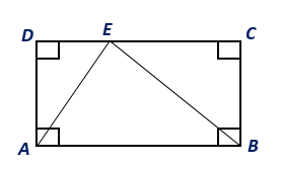Chapter 8.CT, Problem 4CTElementary Geometry For College St...

7th Edition
Alexander + 2 others
ISBN: 9781337614085

Solutions

Chapter
SectionElementary Geometry For College St...

7th Edition
Alexander + 2 others
ISBN: 9781337614085
Textbook Problem

If the area of rectangle ABCD is 46 cm2, find the area of ∆ ABE.To determine

To find:

The area of the triangle ABE.

Explanation

Given:

The area of rectangle ABCD is 46 cm2.

Postulate:

1. The area A of a rectangle whose base has length b and whose altitude has the length h is given by A=bh.

2. The area A of a triangle whose base has length b and whose altitude has the length h is given by A=12bh

Still sussing out bartleby?

Check out a sample textbook solution.

See a sample solution

The Solution to Your Study Problems

Bartleby provides explanations to thousands of textbook problems written by our experts, many with advanced degrees!

Get Started

Place the following set of n = 20 scores in a frequency distribution table.

Essentials of Statistics for The Behavioral Sciences (MindTap Course List)

In Problems 49-54, use properties of definite integrals. 49. How does compare with ?

Mathematical Applications for the Management, Life, and Social Sciences

Let f(x) = 1x+1 and g(x) = x2 + 1. Find the rules for (a) f + g, (b) fg (c) f t, and (d) g f.

Applied Calculus for the Managerial, Life, and Social Sciences: A Brief Approach

In Exercises 1-4, simplify the expression. x316xx3+2x28x

Calculus: An Applied Approach (MindTap Course List)